Engineering ToolBox - Resources, Tools and Basic Information for Engineering and Design of Technical Applications!

# Area Moment of Inertia - Typical Cross Sections I

## Typical cross sections and their Area Moment of Inertia.

Area Moment of Inertia or Moment of Inertia for an Area - also known as Second Moment of Area - I, is a property of shape that is used to predict deflection, bending and stress in beams.

• inches4

• mm4
• cm4
• m4

### Converting between Units

• 1 cm4 = 10-8 m4 = 104 mm4
• 1 in4 = 4.16x105 mm4 = 41.6 cm4

#### Example - Convert between Area Moment of Inertia Units

9240 cm4 can be converted to mm4 by multiplying with 104

(9240 cm4) 104 = 9.24 107 mm4

### Area Moment of Inertia (Moment of Inertia for an Area or Second Moment of Area)for bending around the x axis can be expressed as

Ix = ∫ y2 dA                          (1)

where

Ix = Area Moment of Inertia related to the x axis (m4, mm4, inches4)

y = the perpendicular distance from axis x to the element dA (m, mm, inches)

dA = an elemental area (m2, mm2, inches2)

The Moment of Inertia for bending around the y axis can be expressed as

Iy = ∫ x2 dA                          (2)

where

Iy = Area Moment of Inertia related to the y axis (m4, mm4, inches4)

x = the perpendicular distance from axis y to the element dA (m, mm, inches)

### Area Moment of Inertia for typical Cross Sections I

#### Solid Square Cross Section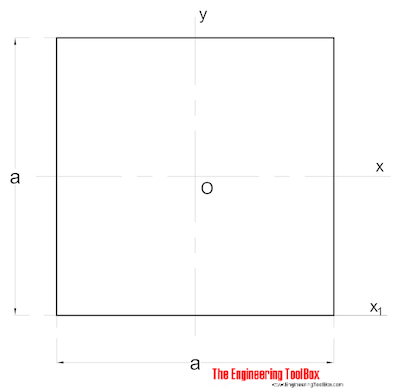The Area Moment of Inertia for a solid square section can be calculated as

Ix = a4 / 12                        (2)

where

a = side (mm, m, in..)

Iy = a4 / 12                         (2b)

#### Solid Rectangular Cross Section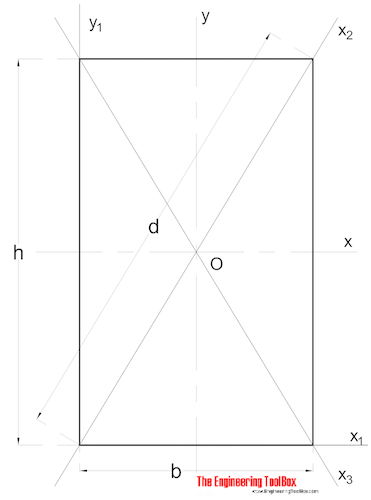The Area Moment of Ineria for a rectangular section can be calculated as

Ix = b h3 / 12                          (3)

where

b = width

h = height

Iy = b3 h / 12                           (3b)

#### Solid Circular Cross Section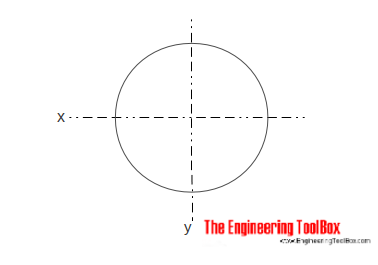The Area Moment of Inertia for a solid cylindrical section can be calculated as

Ix = π r4 / 4

= π d4 / 64                            (4)

where

d = diameter

Iy = π r4 / 4

= π d4 / 64                             (4b)

#### Hollow Cylindrical Cross Section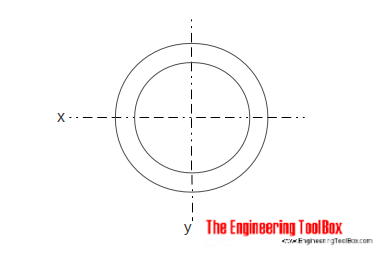The Area Moment of Inertia for a hollow cylindrical section can be calculated as

Ix = π (do4 - di4) / 64                            (5)

where

do = cylinder outside diameter

di = cylinder inside diameter

Iy = π (do4 - di4) / 64                          (5b)

#### Square Section - Diagonal Moments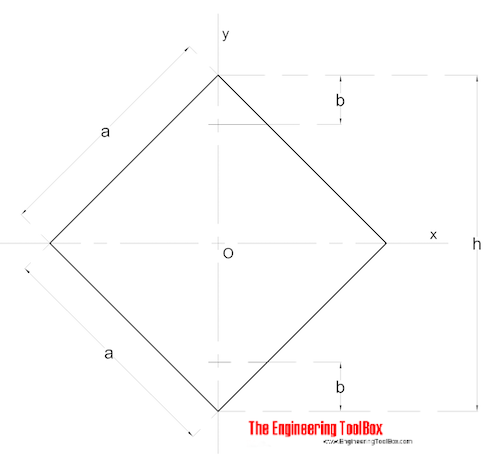The diagonal Area Moments of Inertia for a square section can be calculated as

Ix = Iy = a4 / 12                   (6)

###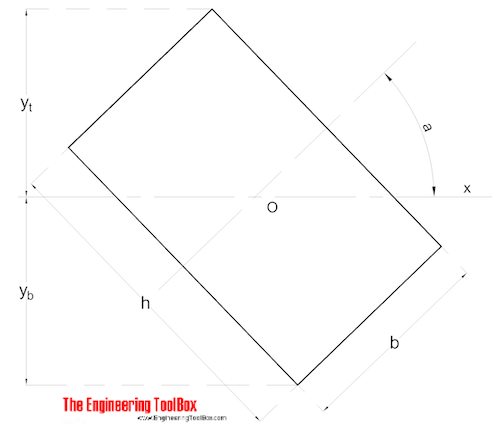Rectangular section and Area of Moment on line through Center of Gravity can be calculated as

Ix = (b h / 12) (h2 cos2 a + b2 sin2 a)                  (7)

#### Symmetrical Shape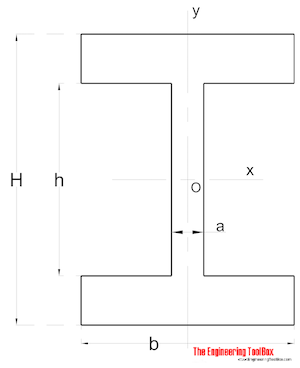Area Moment of Inertia for a symmetrical shaped section can be calculated as

Ix = (a h3 / 12) + (b / 12) (H3 - h3)                       (8)

Iy = (a3 h / 12) + (b3 / 12) (H - h)                       (8b)

#### Nonsymmetrical Shape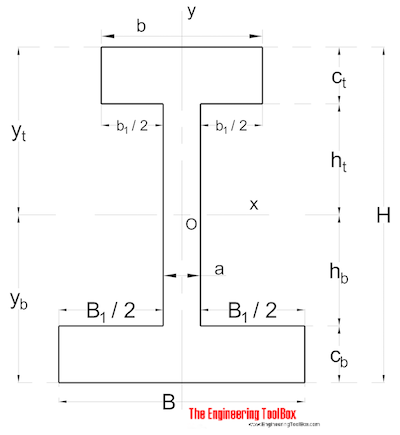Area Moment of Inertia for a non symmetrical shaped section can be calculated as

Ix = (1 / 3) (B yb3 - B1 hb3 + b yt3 - b1 ht3)                       (9)

### Area Moment of Inertia vs. Polar Moment of Inertia vs. Moment of Inertia

• "Area Moment of Inertia" is a property of shape that is used to predict deflection, bending and stress in beams
• "Polar Moment of Inertia" as a measure of a beam's ability to resist torsion - which is required to calculate the twist of a beam subjected to torque
• "Moment of Inertia" is a measure of an object's resistance to change in rotation direction.

### Section Modulus

• the "Section Modulus" is defined as W = I / y, where I is Area Moment of Inertia and y is the distance from the neutral axis to any given fiber

## Related Topics

• ### Beams and Columns

Deflection and stress, moment of inertia, section modulus and technical information of beams and columns.
• ### Mechanics

Forces, acceleration, displacement, vectors, motion, momentum, energy of objects and more.

## Related Documents

• ### American Standard Beams - S Beam

American Standard Beams ASTM A6 - Imperial units.
• ### American Standard Steel C Channels

Dimensions and static parameters of American Standard Steel C Channels
• ### American Wide Flange Beams

American Wide Flange Beams ASTM A6 in metric units.
• ### Area Moment of Inertia - Typical Cross Sections II

Area Moment of Inertia, Moment of Inertia for an Area or Second Moment of Area for typical cross section profiles.
• ### Area Moment of Inertia Converter

Convert between Area Moment of Inertia units.

• ### British Universal Columns and Beams

Properties of British Universal Steel Columns and Beams.
• ### Cantilever Beams - Moments and Deflections

Maximum reaction forces, deflections and moments - single and uniform loads.
• ### Center Mass

Calculate position of center mass.
• ### Center of Gravity

A body and the center of gravity.
• ### Euler's Column Formula

Calculate buckling of columns.
• ### HE-A Steel Beams

Properties of HE-A profiled steel beams.
• ### HE-B Steel Beams

Properties of HE-B profiled steel beams.
• ### HE-M Steel Beams

Properties of HE-M profile steel beams.
• ### Mass Moment of Inertia

The Mass Moment of Inertia vs. mass of object, it's shape and relative point of rotation - the Radius of Gyration.
• ### Mild Steel - Square Bars Weight

Typical weights of mild steel square bars.
• ### Normal Flange I-Beams

Properties of normal flange I profile steel beams.
• ### Pipe Formulas

Pipe and Tube Equations - moment of inertia, section modulus, traverse metal area, external pipe surface and traverse internal area - imperial units
• ### Radius of Gyration in Structural Engineering

Radius of gyration describes the distribution of cross sectional area in columns around their centroidal axis.
• ### Section Modulus - Unit Converter

Convert between Elastic Section Modulus units.
• ### Steel Angles - Equal Legs

Dimensions and static parameters of steel angles with equal legs - metric units.
• ### Steel Angles - Unequal Legs

Dimensions and static parameters of steel angles with unequal legs - imperial units.
• ### Steel Angles - Unequal Legs

Dimensions and static parameters of steel angles with unequal legs - metric units.
• ### Structural Lumber - Section Sizes

Basic size, area, moments of inertia and section modulus for timber - metric units.
• ### Structural Lumber - Properties

Properties of structural lumber.
• ### Three-Hinged Arches - Continuous and Point Loads

Support reactions and bending moments.
• ### W-Beams - American Wide Flange Beams

Dimensions of American Wide Flange Beams ASTM A6 (or W-Beams) - Imperial units.
• ### Weight of Beams - Stress and Strain

Stress and deformation of vertical beams due to own weight.

## Engineering ToolBox - SketchUp Extension - Online 3D modeling!

Add standard and customized parametric components - like flange beams, lumbers, piping, stairs and more - to your Sketchup model with the Engineering ToolBox - SketchUp Extension - enabled for use with older versions of the amazing SketchUp Make and the newer "up to date" SketchUp Pro . Add the Engineering ToolBox extension to your SketchUp Make/Pro from the Extension Warehouse !

We don't collect information from our users. More about

## Citation

• The Engineering ToolBox (2008). Area Moment of Inertia - Typical Cross Sections I. [online] Available at: https://www.engineeringtoolbox.com/area-moment-inertia-d_1328.html [Accessed Day Month Year].

Modify the access date according your visit.

9.29.12